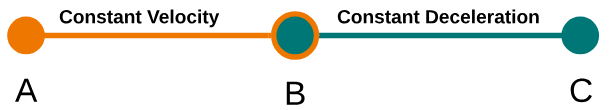# Problem: An inattentive driver is traveling 18.0 m/s when he notices a red light ahead. His car is capable of decelerating at a rate of 3.61 m/s2 .If it takes him 0.210 s to get the brakes on and he is 67.0 m from the intersection when he sees the light, will he be able to stop in time?

###### FREE Expert Solution

This problem starts with a vehicle traveling at some constant vel-ocity and the driver noticing an obstacle or danger ahead. There's a time period after the driver notices the problem where the vehicle continues with a constant velocity, then the driver brakes and the vehicle has a constant deceleration. Whenever we have a problem like this, we'll follow these steps:

1. Break the problem into different intervals. We'll label the two ends of each interval with letters (A, B, C...) It can also help to draw a diagram, as you might have seen in some of our videos!
2. Identify the target variable for the problem.
3. Identify the known variables for each interval.
4. Choose the UAM equations that relate the known variables to the target variable.
5. Solve for the target.

The four UAM (kinematics) equations are:

For problems that involve a reaction time and stopping distance, we'll always have two intervals for Step 1, as shown below:We'll also use a standard coordinate system where the +x-axis points in the direction of travel. That means a is negative, since the acceleration is in the opposite direction (slowing down).

96% (185 ratings)###### Problem Details

An inattentive driver is traveling 18.0 m/s when he notices a red light ahead. His car is capable of decelerating at a rate of 3.61 m/s2 .

If it takes him 0.210 s to get the brakes on and he is 67.0 m from the intersection when he sees the light, will he be able to stop in time?